Two forces with equal magnitudes F act on a body and the magnitude of the resultant force is F/3. The angle between the two forces is

(1) ${\mathrm{cos}}^{-1}\left(-\frac{17}{18}\right)$

(2) ${\mathrm{cos}}^{-1}\left(-\frac{1}{3}\right)$

(3) ${\mathrm{cos}}^{-1}\left(\frac{2}{3}\right)$

(4) ${\mathrm{cos}}^{-1}\left(\frac{8}{9}\right)$

Concept Questions :-

Newton laws
High Yielding Test Series + Question Bank - NEET 2020

Difficulty Level:

Three forces starts acting simultaneously on a particle moving with velocity $\stackrel{\to }{v}.$ These forces are represented in magnitude and direction by the three sides of a triangle ABC (as shown). The particle will now move with velocity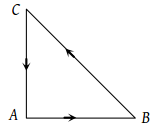(1) $\stackrel{\to }{v}$ remaining unchanged

(2) Less than $\stackrel{\to }{v}$

(3) Greater than $\stackrel{\to }{v}$

(4) $\stackrel{\to }{v}$ in the direction of the largest force BC

Concept Questions :-

Application of laws
High Yielding Test Series + Question Bank - NEET 2020

Difficulty Level:

Two blocks are connected by a string as shown in the diagram. The upper block is hung by another string. A force F applied on the upper string produces an acceleration of 2 m/s2 in the upward direction in both the blocks. If T and T’ be the tensions in the two parts of the string, then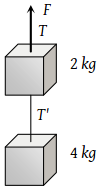(1) T = 70.8 N and T’ = 47.2 N

(2) T = 58.8 N and T’ = 47.2 N

(3) T = 70.8 N and T’ = 58.8 N

(4) T = 70.8 N and T’ = 40.2 N

Concept Questions :-

Application of laws
High Yielding Test Series + Question Bank - NEET 2020

Difficulty Level:

If two forces of 5 N each are acting along X and Y axes, then the magnitude and direction of resultant is

(1) $5\sqrt{2},\text{\hspace{0.17em}\hspace{0.17em}}\pi /3$

(2) $5\sqrt{2},\text{\hspace{0.17em}\hspace{0.17em}}\pi /4$

(3) $-5\sqrt{2},\text{\hspace{0.17em}\hspace{0.17em}}\pi /3$

(4) $-5\sqrt{2},\text{\hspace{0.17em}\hspace{0.17em}}\pi /4$

Concept Questions :-

Types of forces
High Yielding Test Series + Question Bank - NEET 2020

Difficulty Level:

A block is kept on a frictionless inclined surface with angle of inclination 'α'. The incline is given an acceleration 'a' to keep the block stationary. Then a is equal to

(1) g

(2) g tan α

(3) g / tan α

(4) g cosec α

Concept Questions :-

Pseudo force
High Yielding Test Series + Question Bank - NEET 2020

Difficulty Level:

A block of mass M is pulled along a horizontal frictionless surface by a rope of mass m. If a force P is applied at the free end of the rope, the force exerted by the rope on the block will be

(1) P

(2) $\frac{Pm}{M+m}$

(3) $\frac{PM}{M+m}$

(4) $\frac{Pm}{M-m}$

Concept Questions :-

Application of laws
High Yielding Test Series + Question Bank - NEET 2020

Difficulty Level:

Three equal weights A, B and C of mass 2 kg each are hanging on a string passing over a fixed frictionless pulley as shown in the figure The tension in the string connecting weights B and C is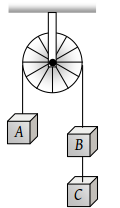(1) Zero

(2) 13 N

(3) 3.3 N

(4) 19.6 N

Concept Questions :-

Application of laws
High Yielding Test Series + Question Bank - NEET 2020

Difficulty Level:

Two masses of 4 kg and 5 kg are connected by a string passing through a frictionless pulley and are kept on a frictionless table as shown in the figure. The acceleration of 5 kg mass is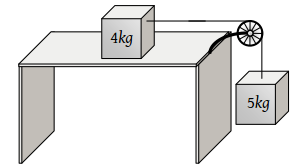(1) 49 m/s2

(2) 5.44 m/s2

(3) 19.5 m/s2

(4) 2.72 m/s2

Concept Questions :-

Application of laws
High Yielding Test Series + Question Bank - NEET 2020

Difficulty Level:

Two masses 2 kg and 3 kg are attached to the end of the string passed over a pulley fixed at the top. The tension and acceleration are

(1) $\frac{7g}{8};\text{\hspace{0.17em}}\frac{g}{8}$

(2) $\frac{21g}{8};\text{\hspace{0.17em}}\frac{g}{8}$

(3) $\frac{21g}{8};\text{\hspace{0.17em}}\frac{g}{5}$

(4) $\frac{12g}{5};\text{\hspace{0.17em}}\frac{g}{5}$

Concept Questions :-

Application of laws
High Yielding Test Series + Question Bank - NEET 2020

Difficulty Level:

Three blocks A, B and C weighing 1, 8 and 27 kg respectively are connected as shown in the figure with an inextensible string and are moving on a smooth surface. T3 is equal to 36 N. Then T2 is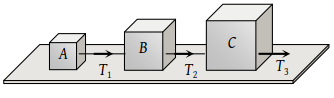(1) 18 N

(2) 9 N

(3) 3.375 N

(4) 1.25 N

Concept Questions :-

Newton laws